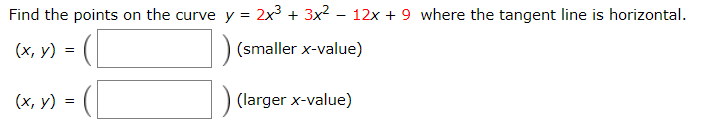# Find the points on the curve y = 2x3 + 3x2 – 12x + 9 where the tangent line is horizontal.(x, y)(smaller x-value)(larger x-value)(x, y)

Question
22 viewshelp_outlineImage TranscriptioncloseFind the points on the curve y = 2x3 + 3x2 – 12x + 9 where the tangent line is horizontal. (x, y) (smaller x-value) (larger x-value) (x, y) fullscreen
check_circle

Step 1

According to the given information it is required to calculate the points on the curve where tangent is horizontal which means tangent is parallel to x axis that is dy/dx = 0

The given curve is:

Step 2

Now, find the derivative of y with respect to x and put it to zero.

Step 3

Now, put dy/d...

### Want to see the full answer?

See Solution

#### Want to see this answer and more?

Solutions are written by subject experts who are available 24/7. Questions are typically answered within 1 hour.*

See Solution
*Response times may vary by subject and question.
Tagged in
MathCalculus

### Derivative